# R S Aggarwal Solutions for Class 10 Maths Chapter 6 Coordinate Geometry Exercise 6A

R S Aggarwal Solutions for Class 10 Maths exercise 6A are given here. In this exercise, students will learn about the distance between two points, properties of various types of quadrilaterals and collinearity of three points. Students are advised to practice as many questions of Class 10 Maths Chapter 6 R S Aggarwal Solutions in order to solve all possible questions that will be asked in the final exam.

## Download PDF of R S Aggarwal Solutions for Class 10 Maths Chapter 6 Coordinate Geometry Exercise 6A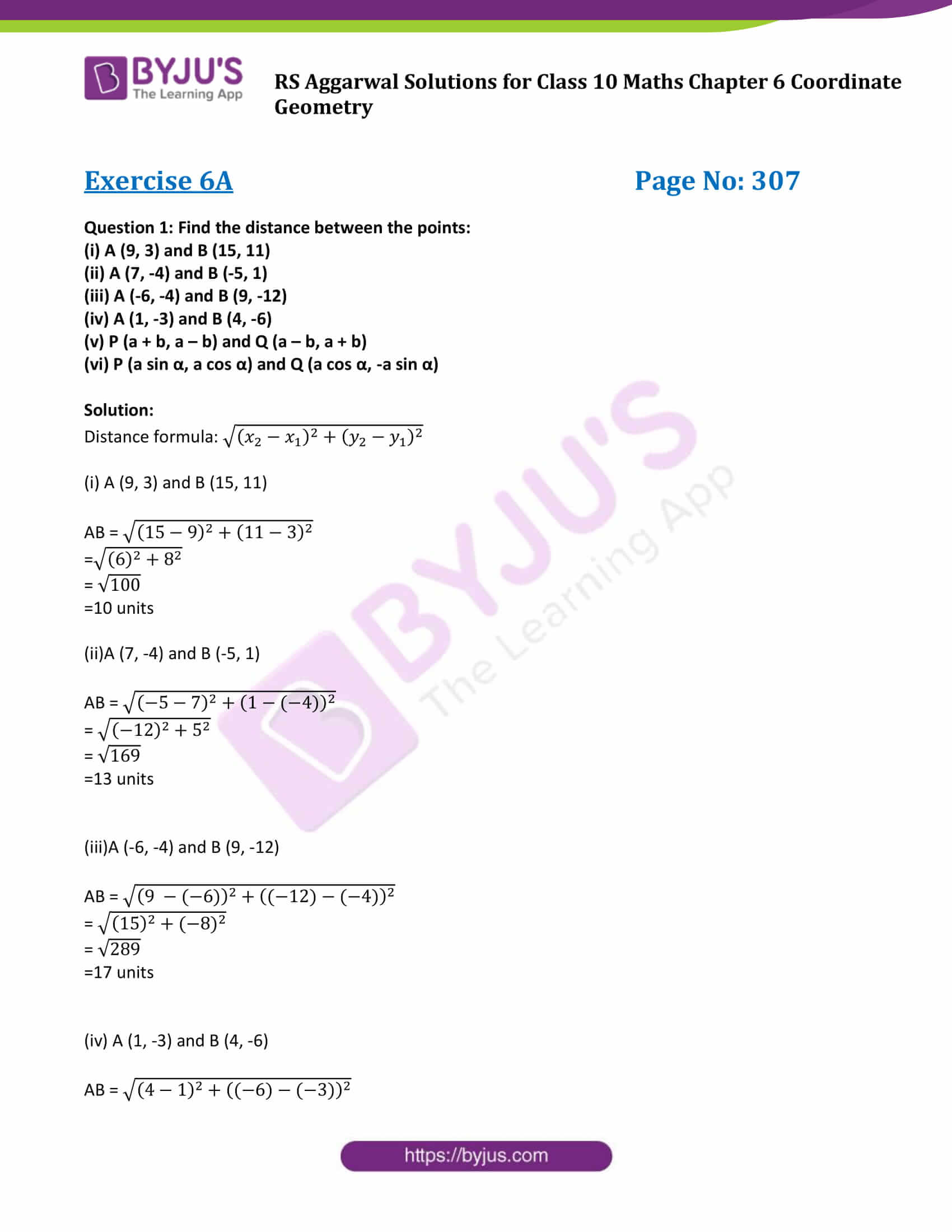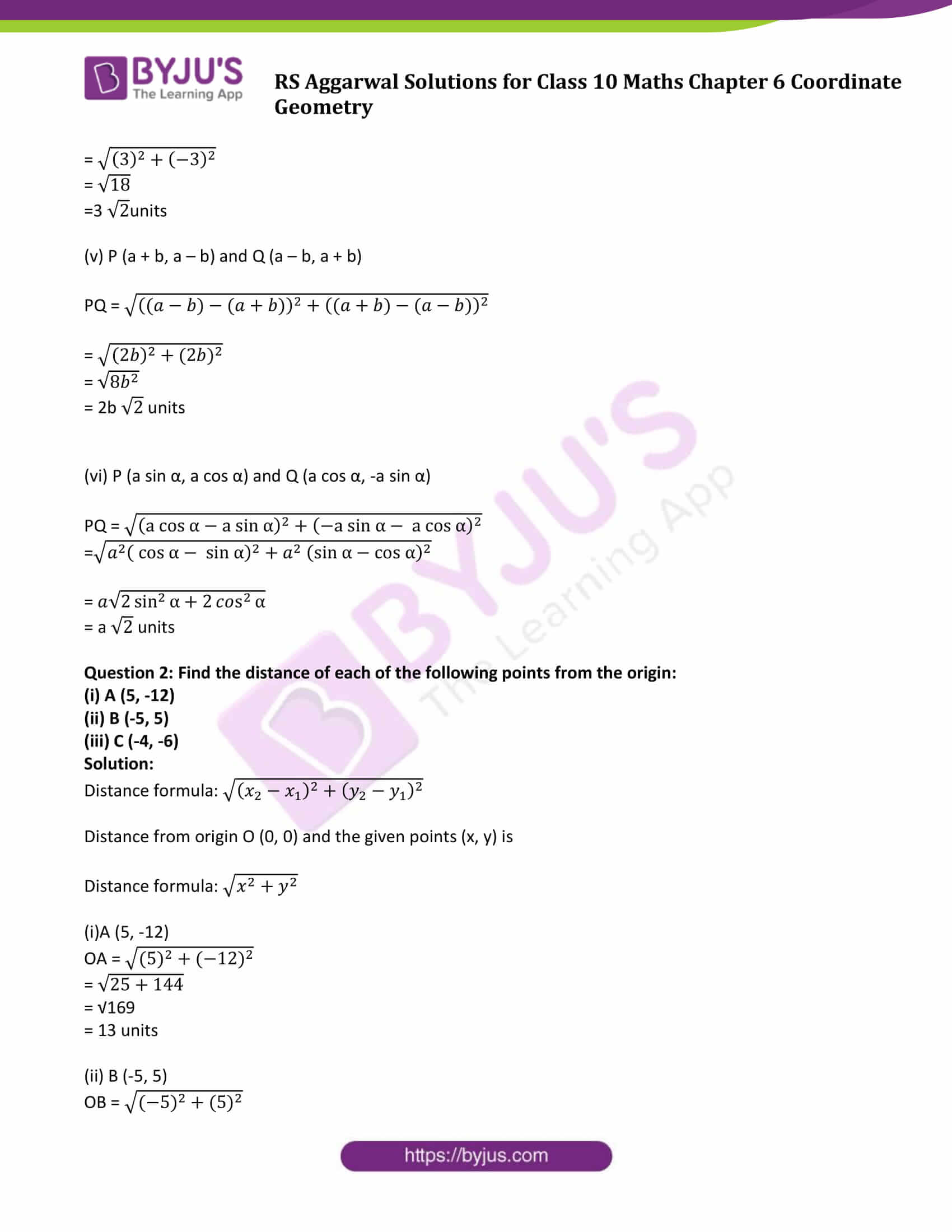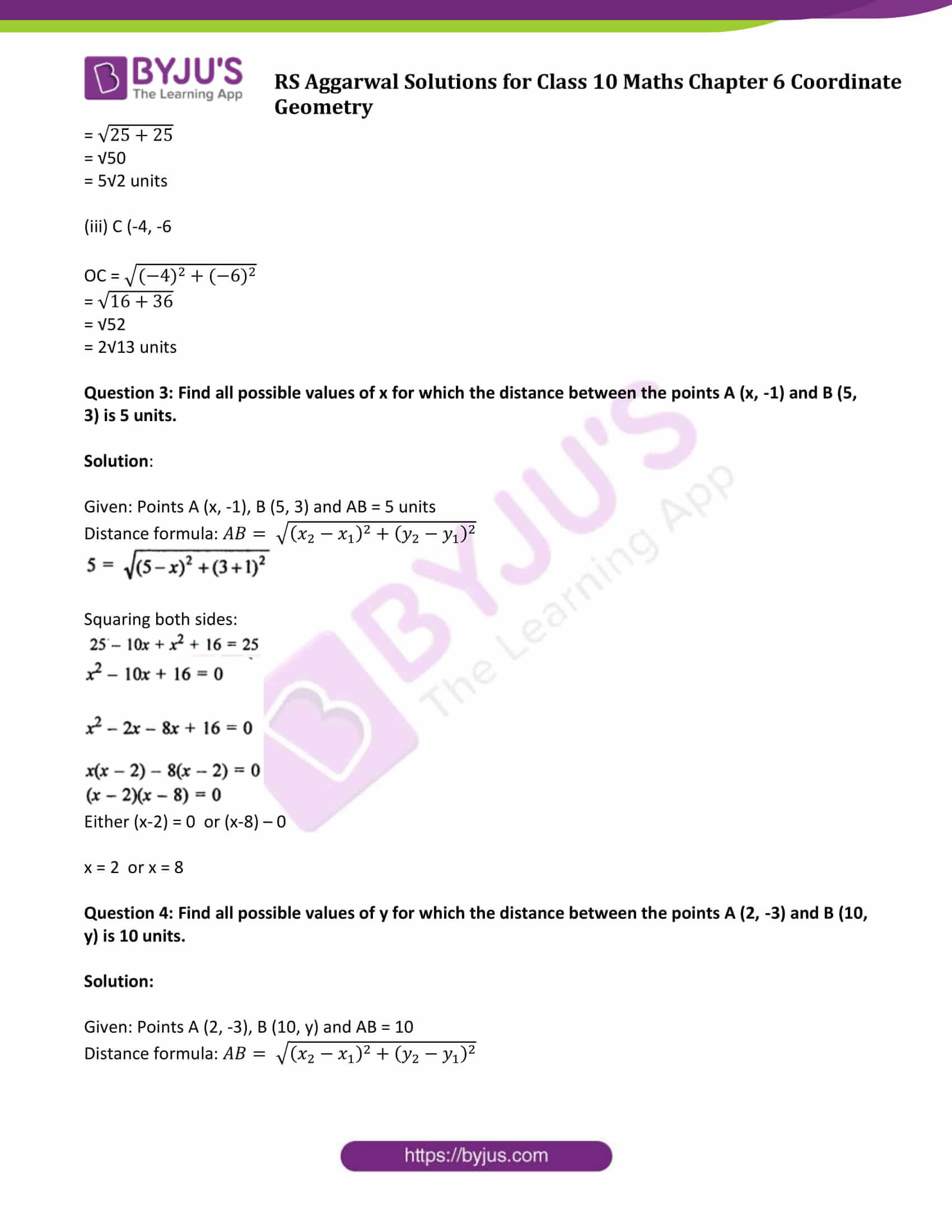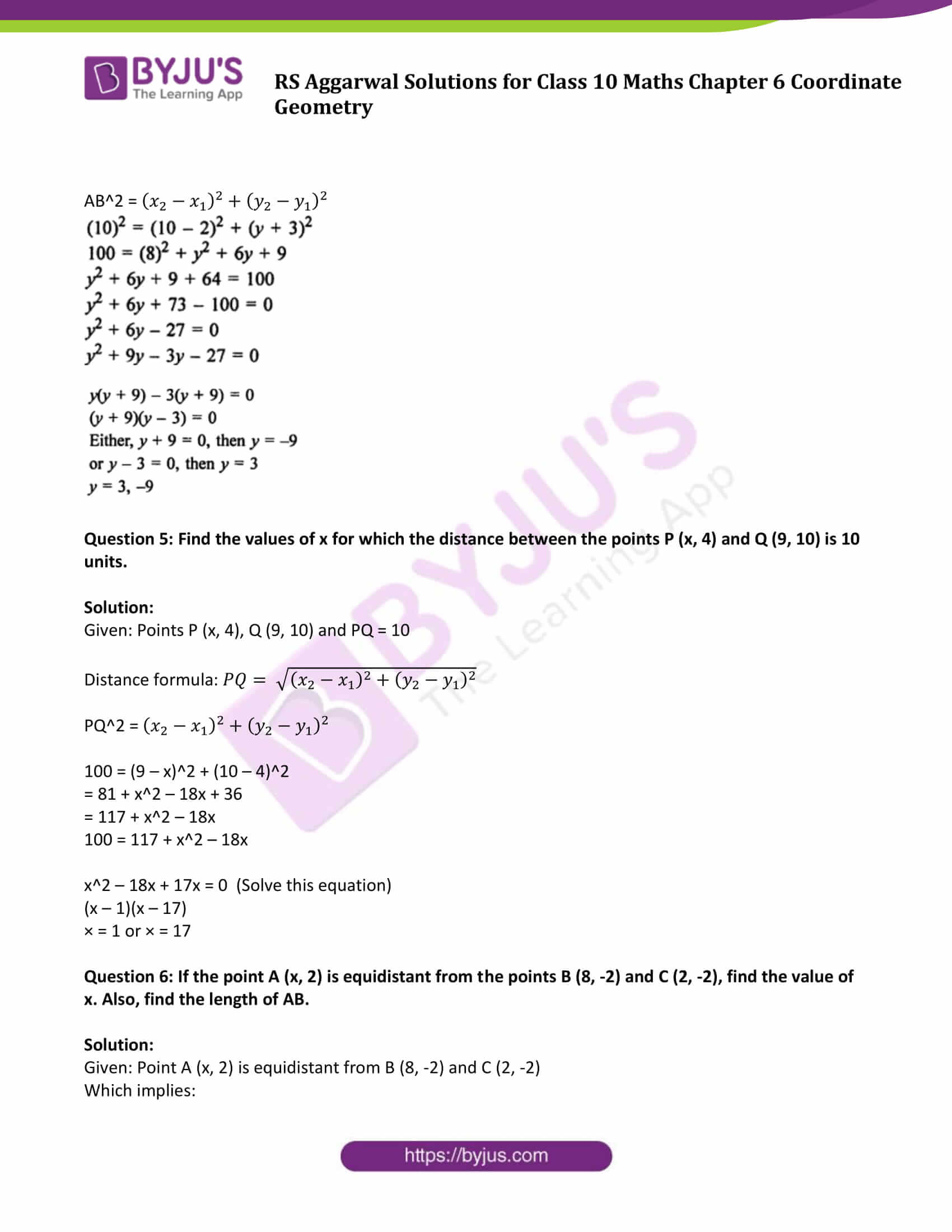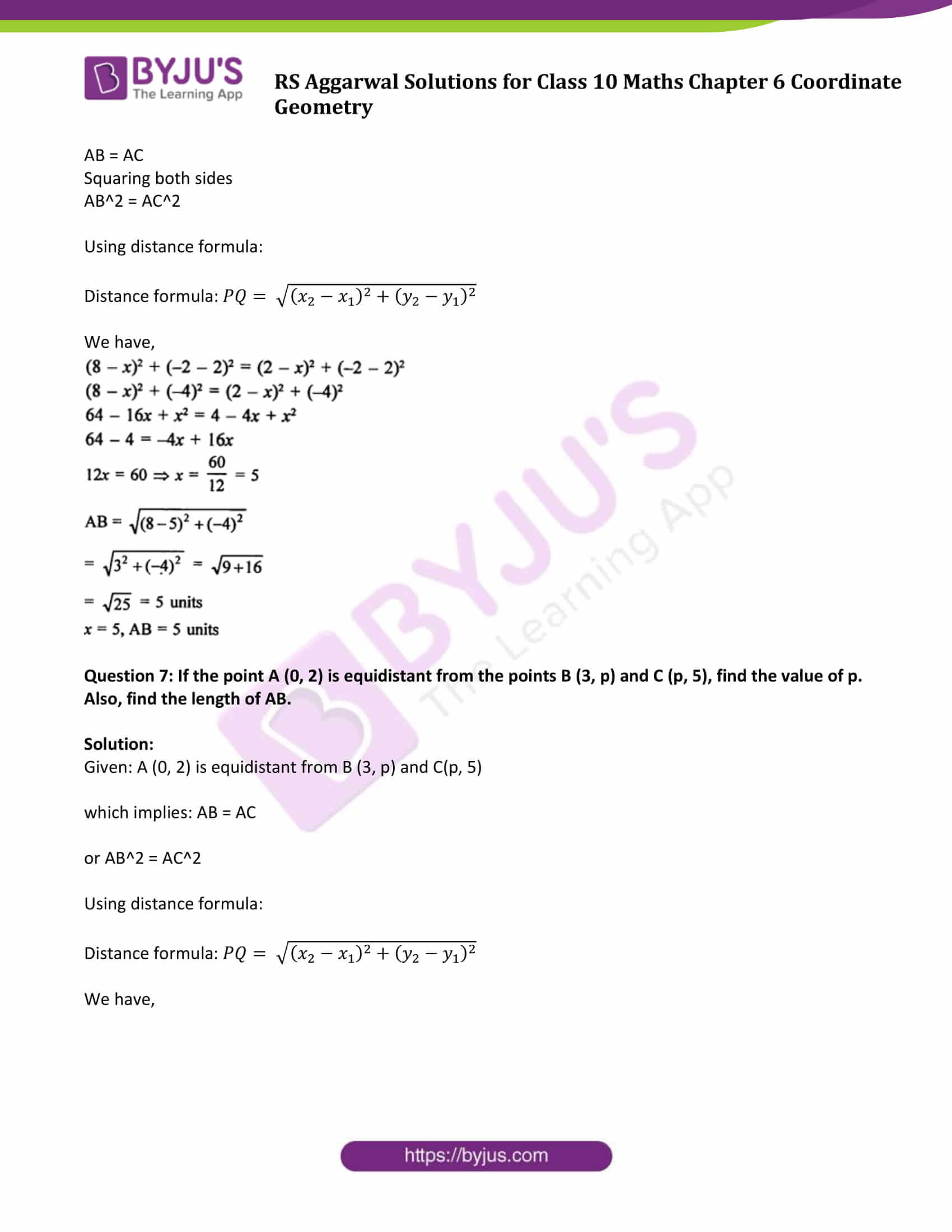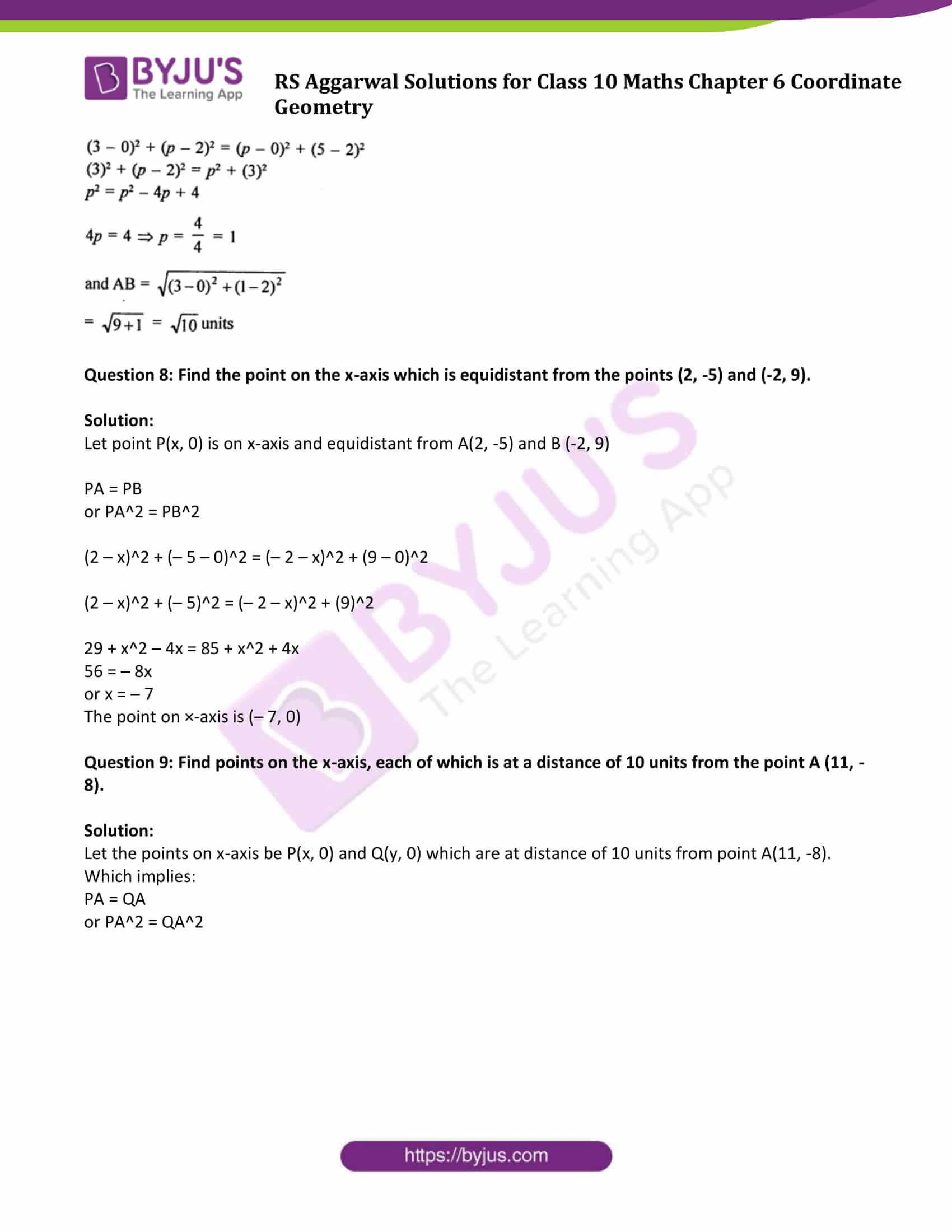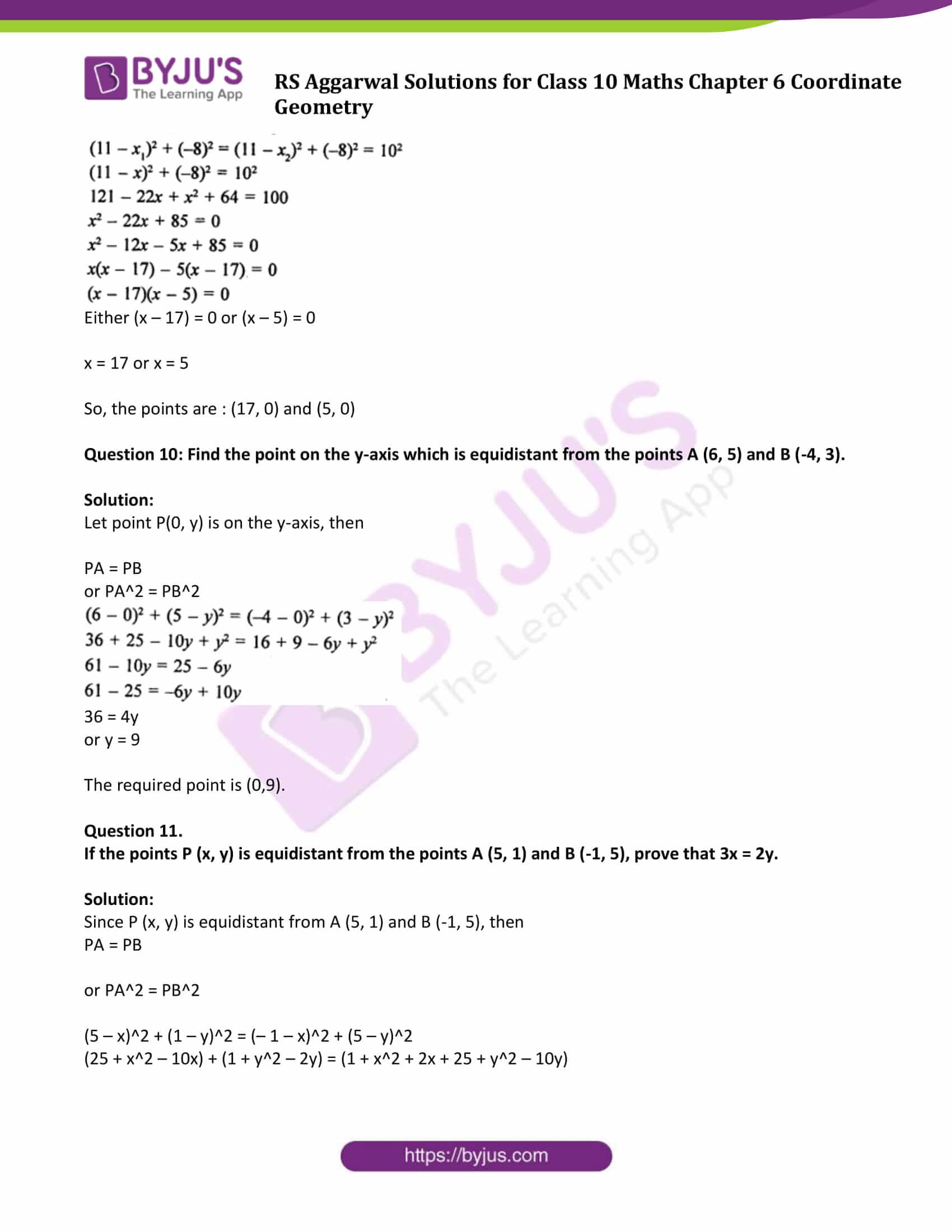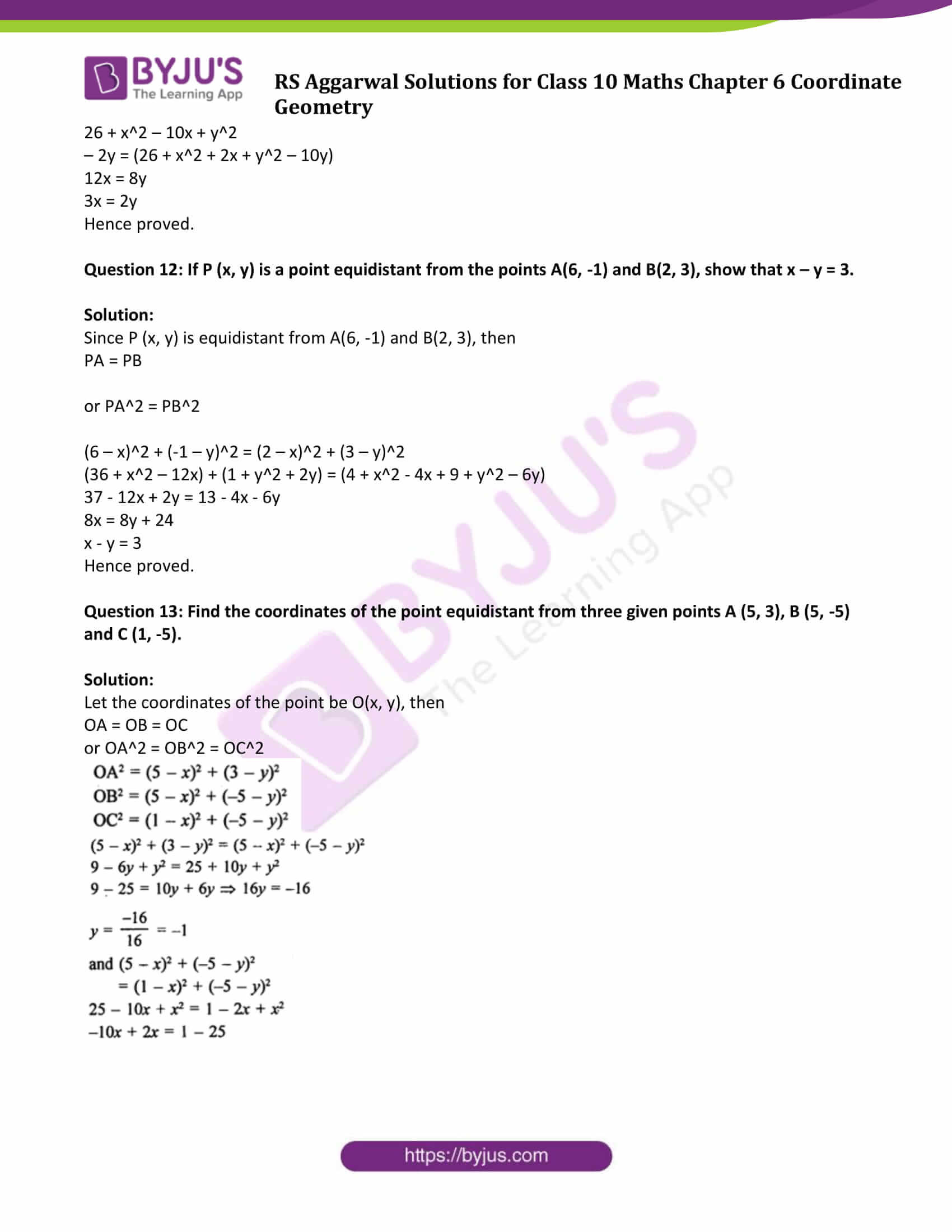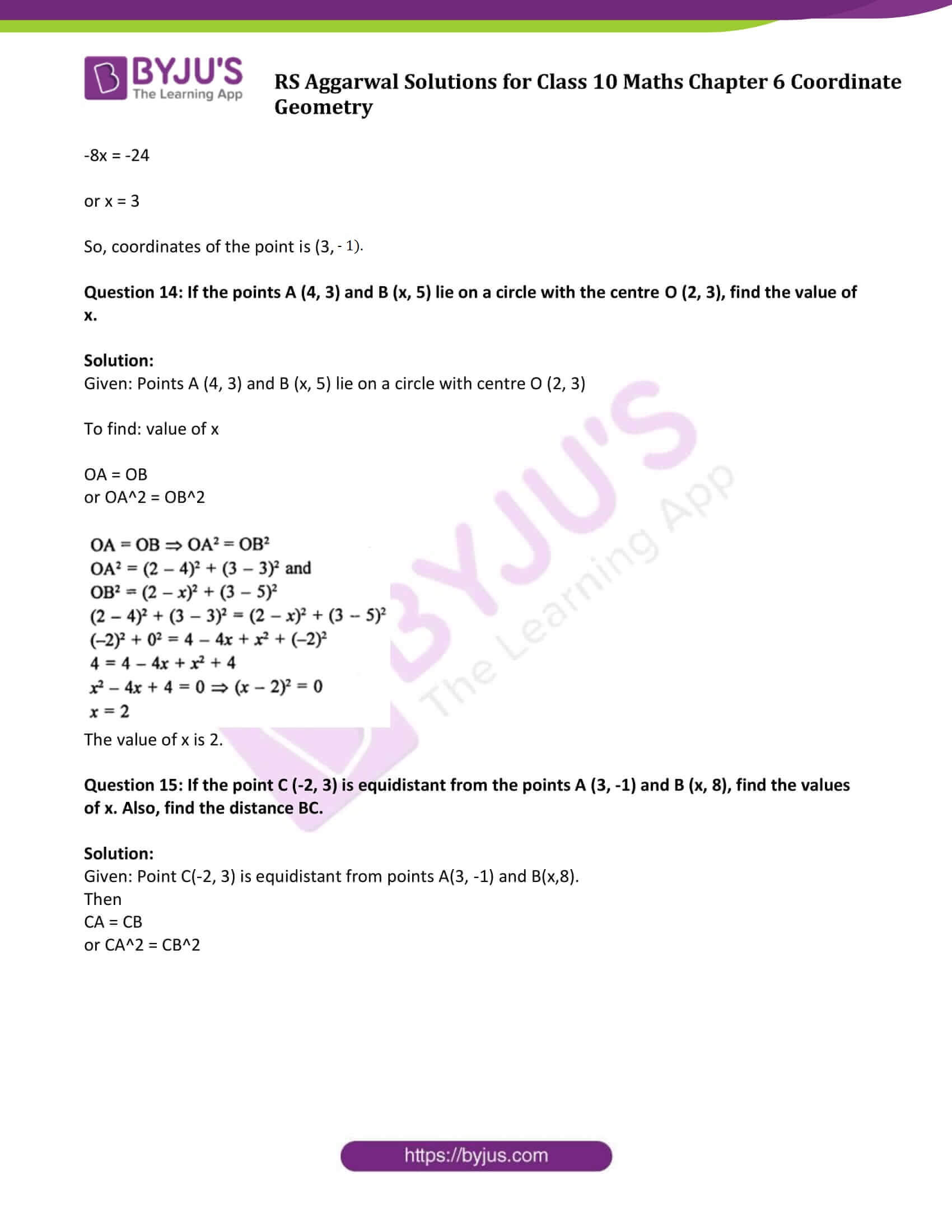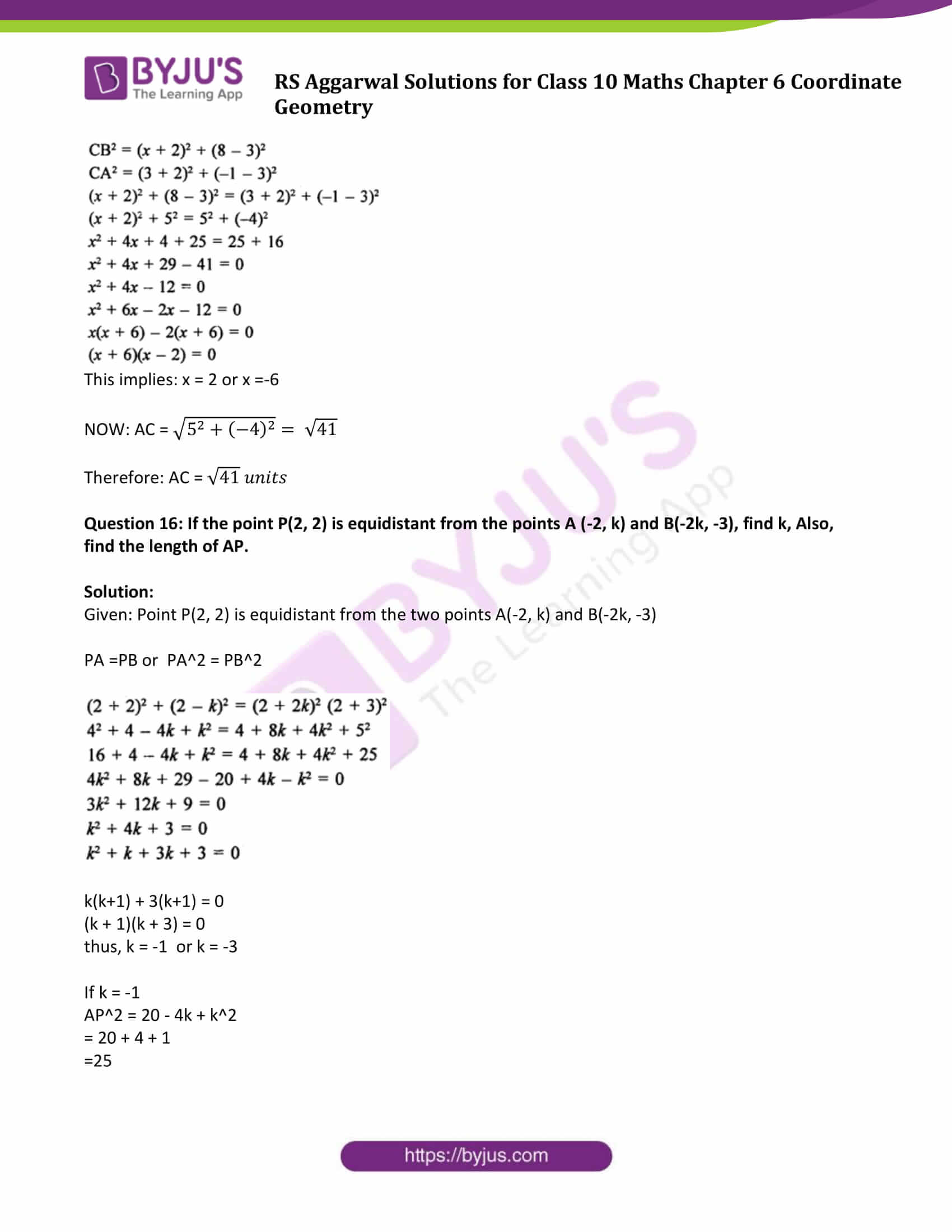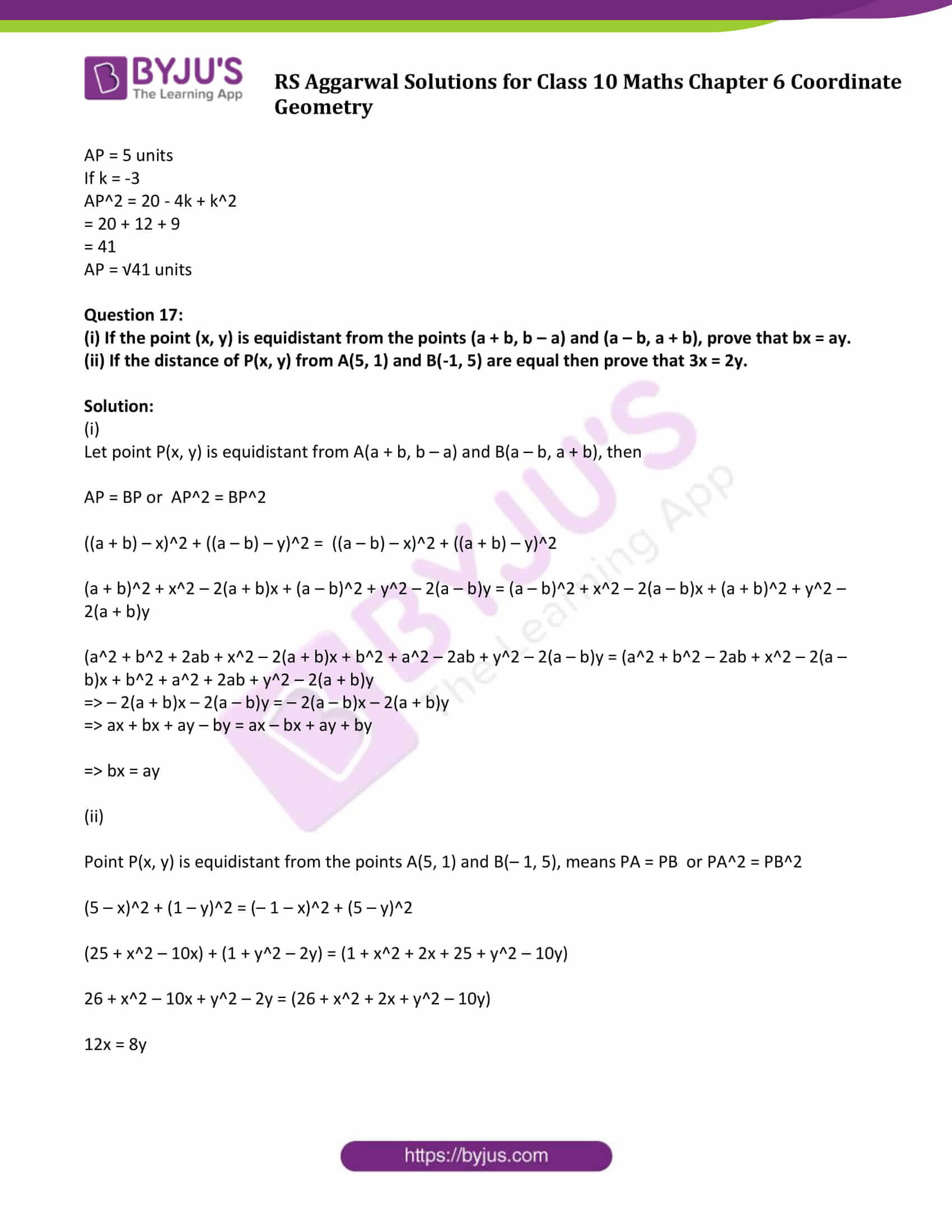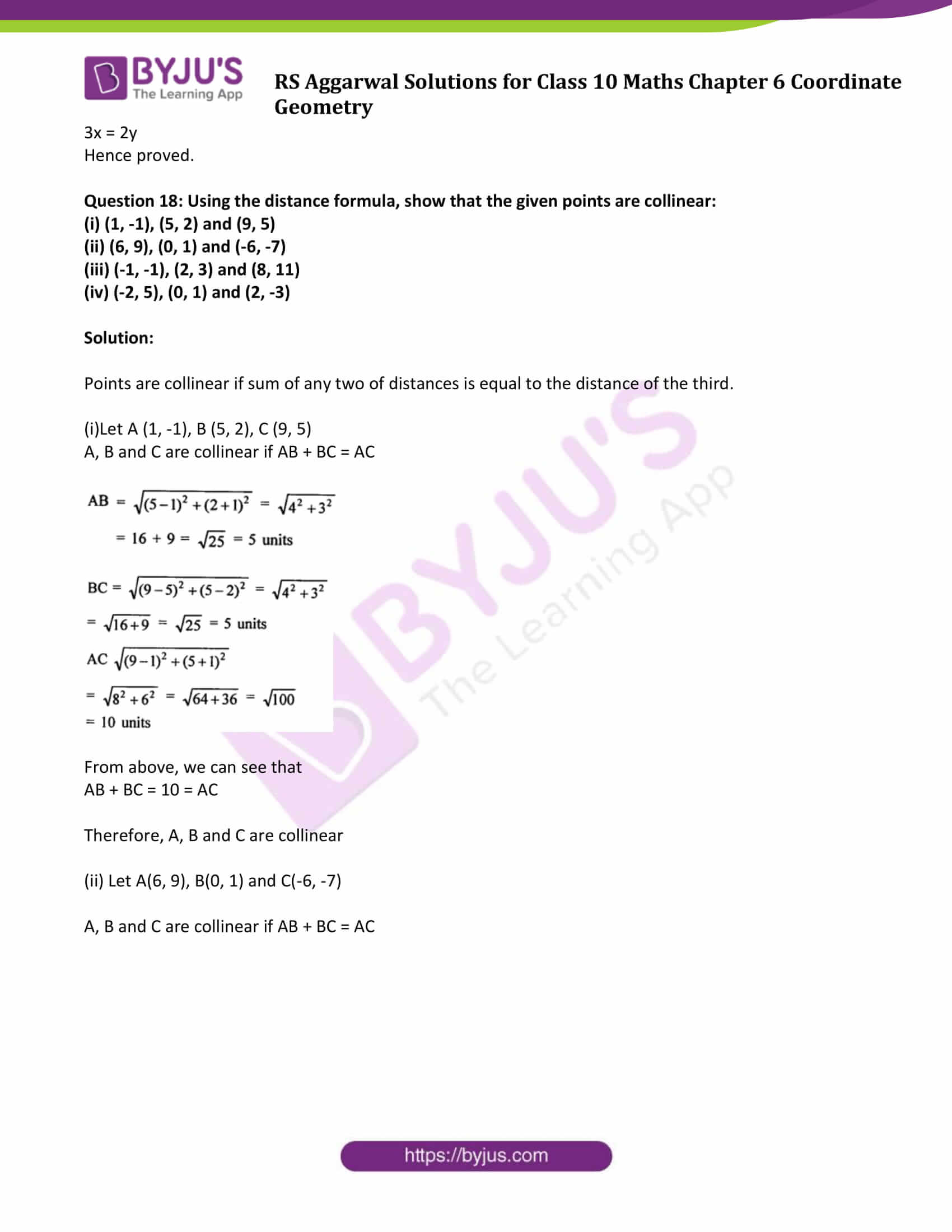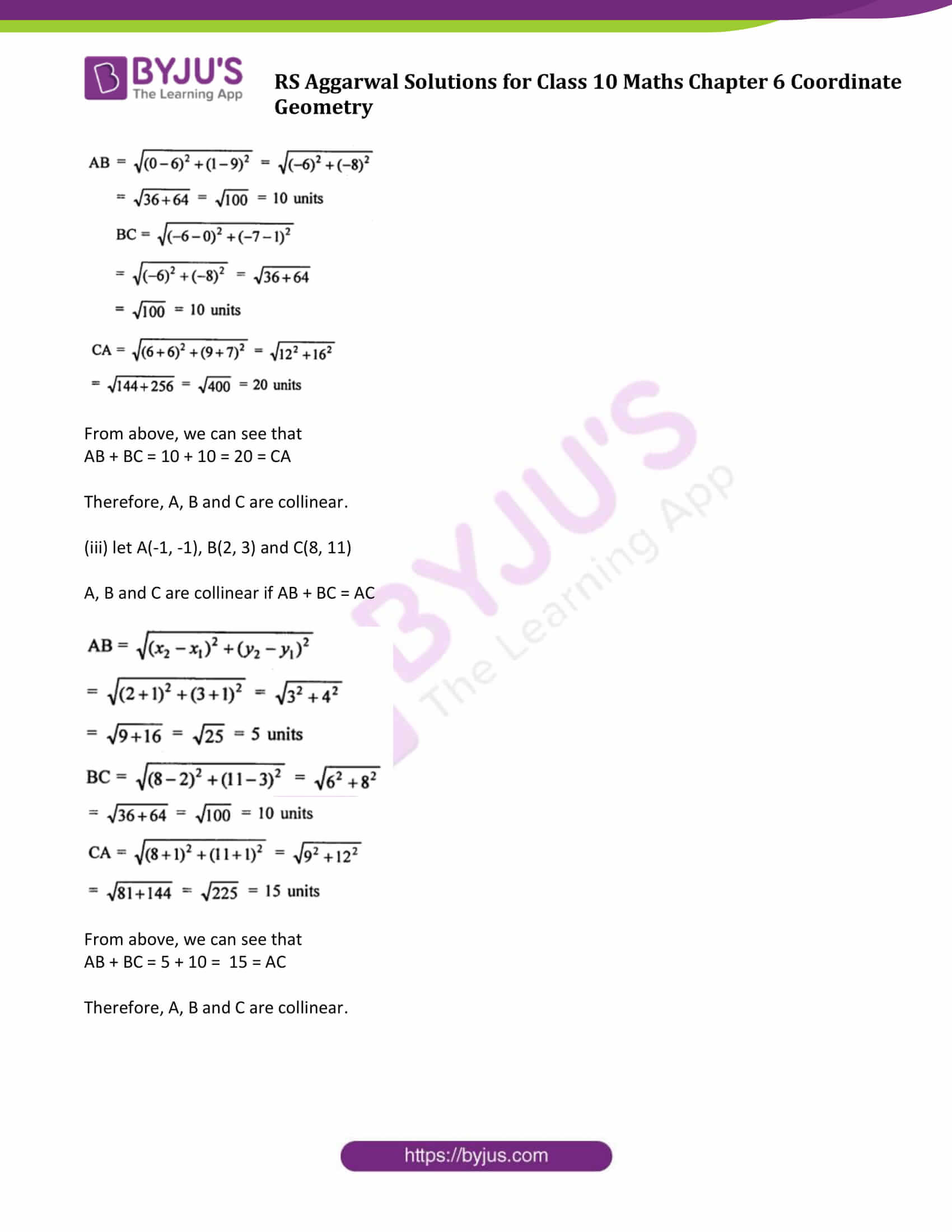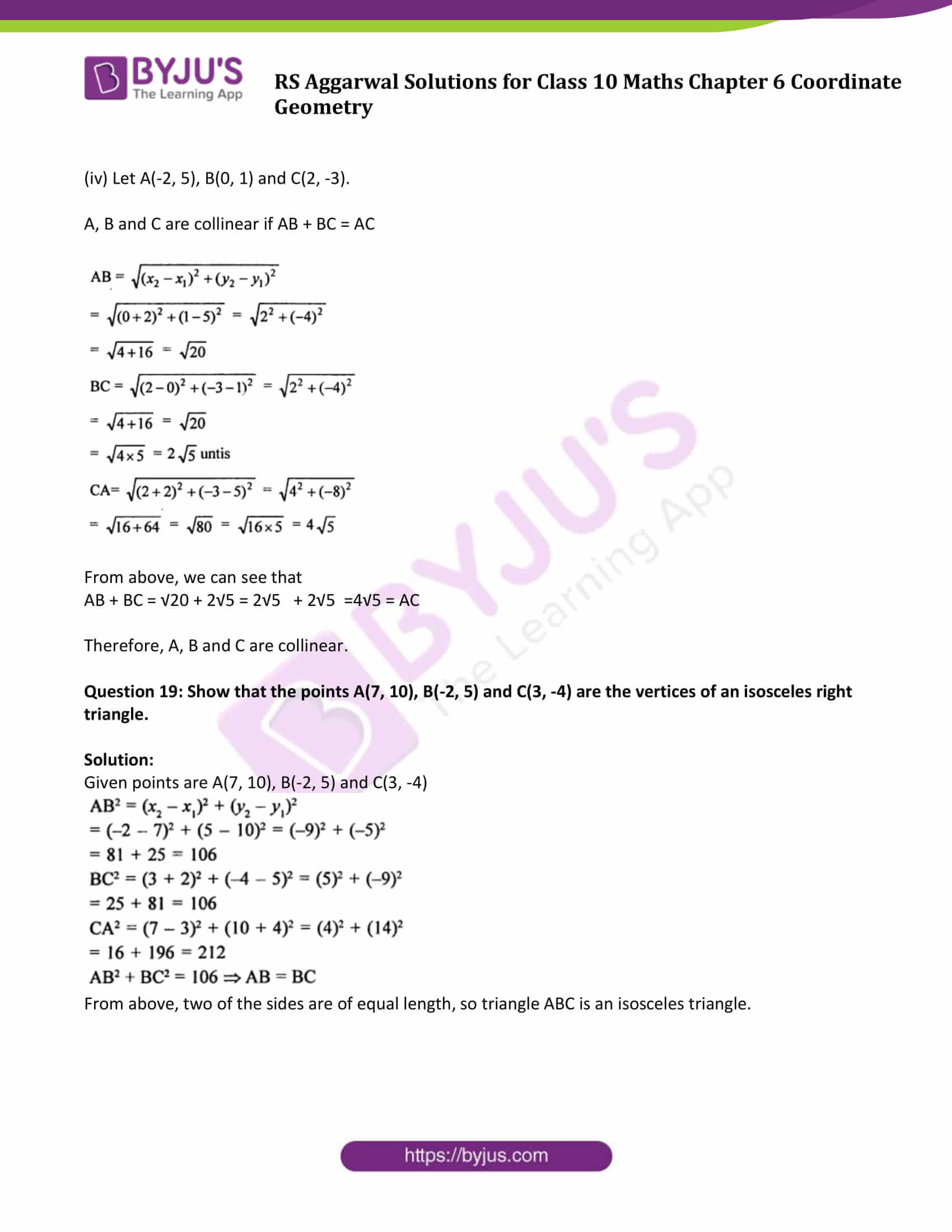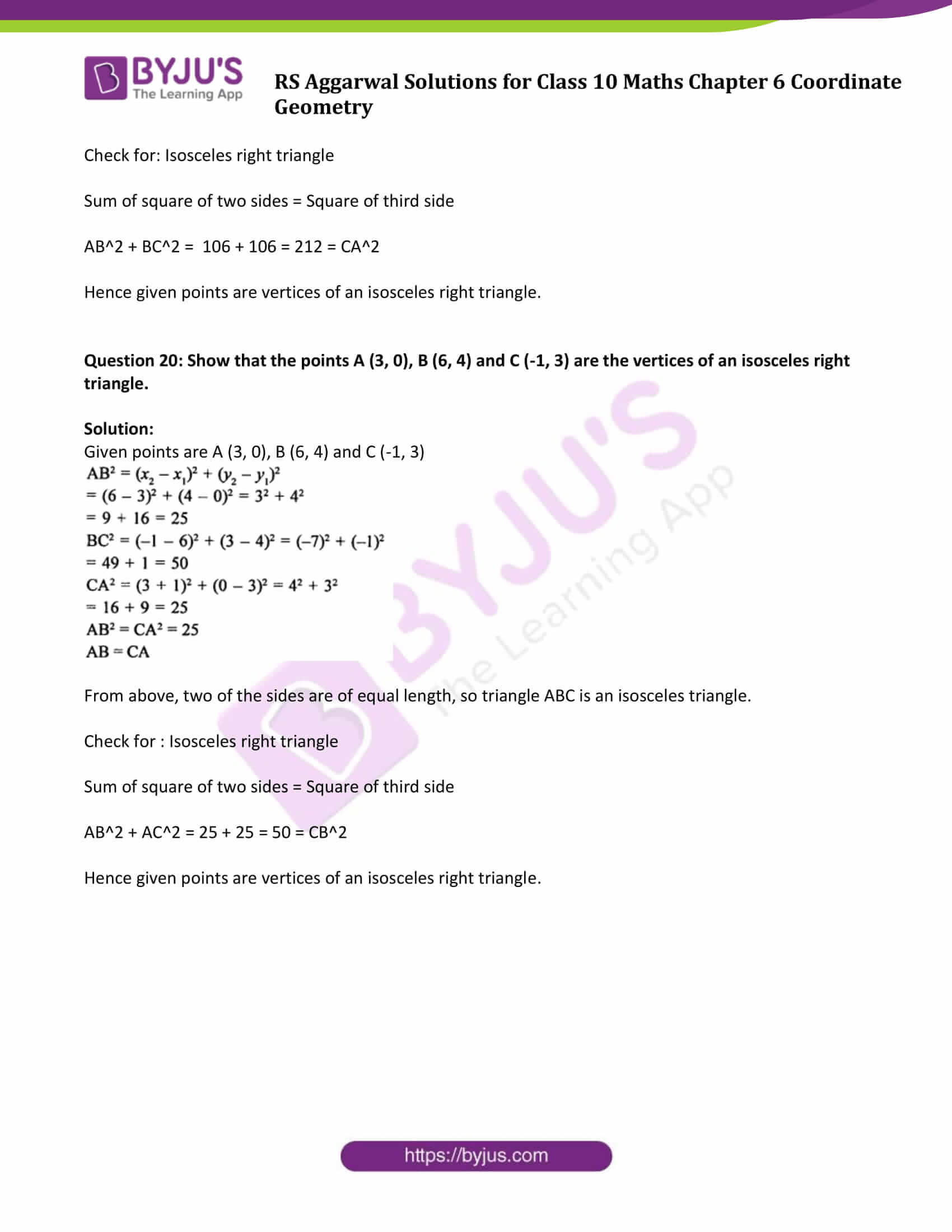### Access other exercise solutions of Class 10 Maths Chapter 6 Coordinate Geometry

Exercise 6B Solutions: 20 Questions (20 Short Answers)

Exercise 6C Solutions: 31 Questions (31 Long Answers)

Exercise 6D Solutions: 17 Questions (12 Short Answers & 5 Long Answers )

## Exercise 6A Page No: 307

Question 1: Find the distance between the points:

(i) A (9, 3) and B (15, 11)

(ii) A (7, -4) and B (-5, 1)

(iii) A (-6, -4) and B (9, -12)

(iv) A (1, -3) and B (4, -6)

(v) P (a + b, a – b) and Q (a – b, a + b)

(vi) P (a sin α, a cos α) and Q (a cos α, -a sin α)

Solution: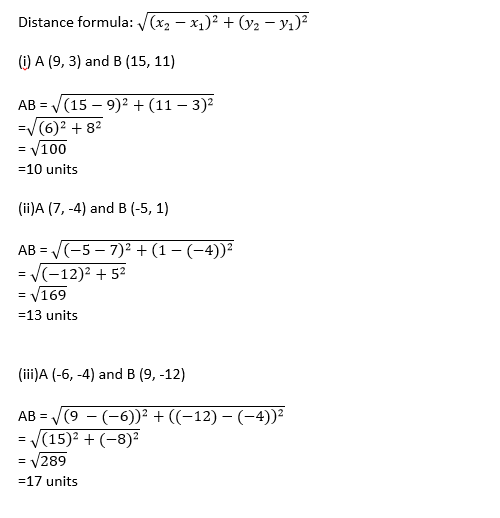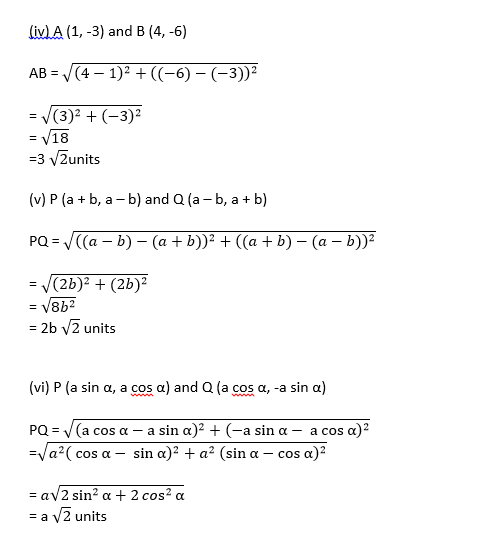Question 2: Find the distance of each of the following points from the origin:

(i) A (5, -12)

(ii) B (-5, 5)

(iii) C (-4, -6)

Solution: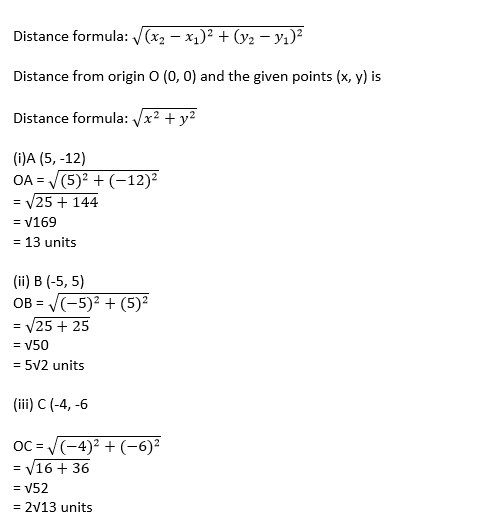Question 3: Find all possible values of x for which the distance between the points A (x, -1) and B (5, 3) is 5 units.

Solution: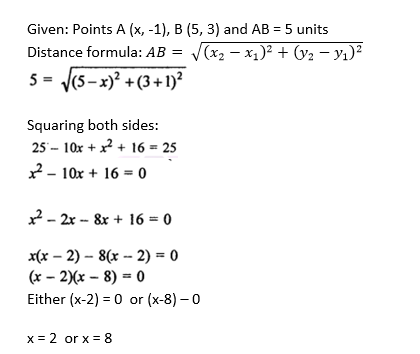Question 4: Find all possible values of y for which the distance between the points A (2, -3) and B (10, y) is 10 units.

Solution: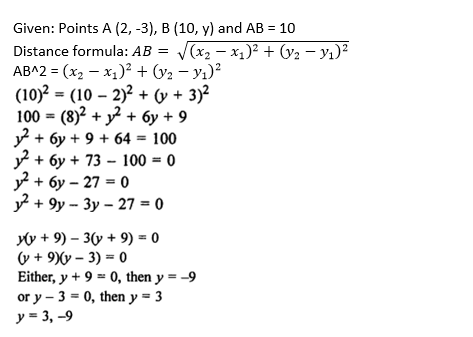Question 5: Find the values of x for which the distance between the points P (x, 4) and Q (9, 10) is 10 units.

Solution: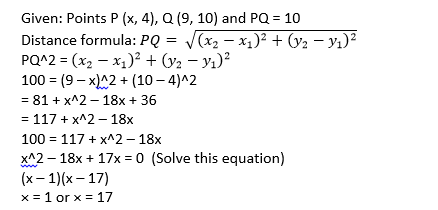Question 6: If the point A (x, 2) is equidistant from the points B (8, -2) and C (2, -2), find the value of x. Also, find the length of AB.

Solution:

Given: Point A (x, 2) is equidistant from B (8, -2) and C (2, -2)

Which implies:

AB = AC

Squaring both sides

AB^2 = AC^2

Using distance formula: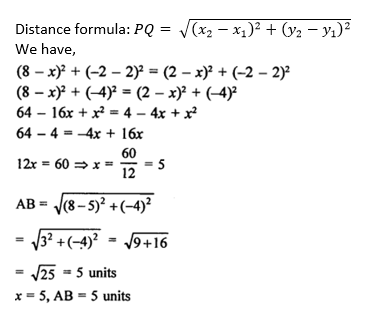Question 7: If the point A (0, 2) is equidistant from the points B (3, p) and C (p, 5), find the value of p. Also, find the length of AB.

Solution: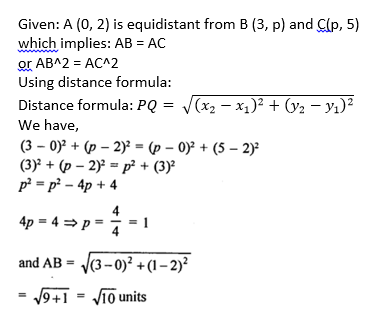Question 8: Find the point on the x-axis which is equidistant from the points (2, -5) and (-2, 9).

Solution:

Let point P(x, 0) is on x-axis and equidistant from A(2, -5) and B (-2, 9)

PA = PB

or PA^2 = PB^2

(2 – x)^2 + (– 5 – 0)^2 = (– 2 – x)^2 + (9 – 0)^2

(2 – x)^2 + (– 5)^2 = (– 2 – x)^2 + (9)^2

29 + x^2 – 4x = 85 + x^2 + 4x

56 = – 8x

or x = – 7

The point on ×-axis is (– 7, 0)

Question 9: Find points on the x-axis, each of which is at a distance of 10 units from the point A (11, -8).

Solution:

Let the points on x-axis be P(x, 0) and Q(y, 0) which are at distance of 10 units from point A(11, -8).

Which implies:

PA = QA

or PA^2 = QA^2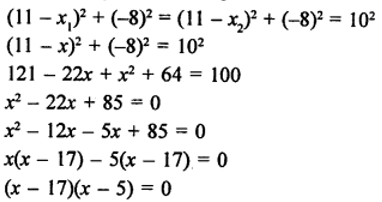Either (x – 17) = 0 or (x – 5) = 0

x = 17 or x = 5

So, the points are : (17, 0) and (5, 0)

Question 10: Find the point on the y-axis which is equidistant from the points A (6, 5) and B (-4, 3).

Solution:

Let point P(0, y) is on the y-axis, then

PA = PB

or PA^2 = PB^2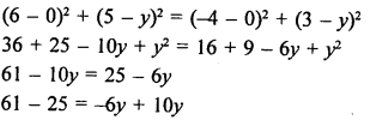36 = 4y

or y = 9

The required point is (0,9).

Question 11.

If the points P (x, y) is equidistant from the points A (5, 1) and B (-1, 5), prove that 3x = 2y.

Solution:

Since P (x, y) is equidistant from A (5, 1) and B (-1, 5), then

PA = PB

or PA^2 = PB^2

(5 – x)^2 + (1 – y)^2 = (– 1 – x)^2 + (5 – y)^2

(25 + x^2 – 10x) + (1 + y^2 – 2y) = (1 + x^2 + 2x + 25 + y^2 – 10y)

26 + x^2 – 10x + y^2 – 2y = (26 + x^2 + 2x + y^2 – 10y)

12x = 8y

3x = 2y

Hence proved.

Question 12: If P (x, y) is a point equidistant from the points A(6, -1) and B(2, 3), show that x – y = 3.

Solution:

Since P (x, y) is equidistant from A(6, -1) and B(2, 3), then

PA = PB

or PA^2 = PB^2

(6 – x)^2 + (-1 – y)^2 = (2 – x)^2 + (3 – y)^2

(36 + x^2 – 12x) + (1 + y^2 + 2y) = (4 + x^2 – 4x + 9 + y^2 – 6y)

37 – 12x + 2y = 13 – 4x – 6y

8x = 8y + 24

x – y = 3

Hence proved.

Question 13: Find the coordinates of the point equidistant from three given points A (5, 3), B (5, -5) and C (1, -5).

Solution:

Let the coordinates of the point be O(x, y), then

OA = OB = OC

or OA^2 = OB^2 = OC^2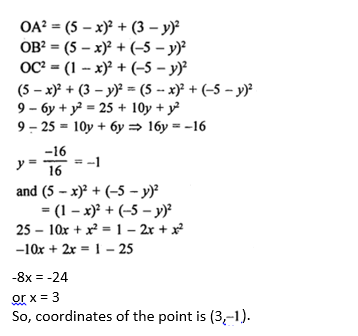Question 14: If the points A (4, 3) and B (x, 5) lie on a circle with the centre O (2, 3), find the value of x.

Solution:

Given: Points A (4, 3) and B (x, 5) lie on a circle with centre O (2, 3)

To find: value of x

OA = OB

or OA^2 = OB^2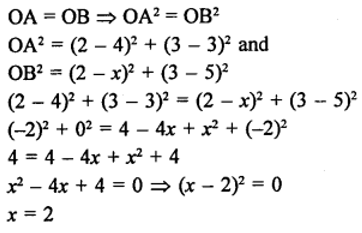The value of x is 2.

Question 15: If the point C (-2, 3) is equidistant from the points A (3, -1) and B (x, 8), find the values of x. Also, find the distance BC.

Solution:

Given: Point C(-2, 3) is equidistant from points A(3, -1) and B(x,8).

Then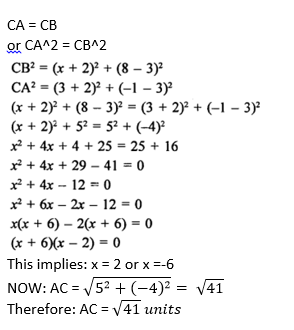Question 16: If the point P(2, 2) is equidistant from the points A (-2, k) and B(-2k, -3), find k, Also, find the length of AP.

Solution:

Given: Point P(2, 2) is equidistant from the two points A(-2, k) and B(-2k, -3)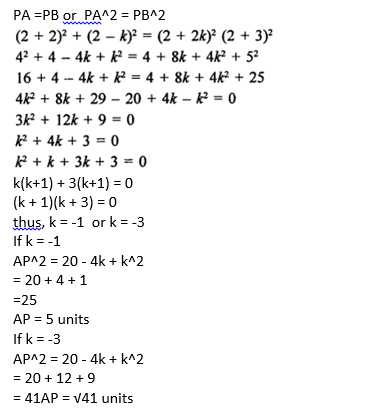Question 17:

(i) If the point (x, y) is equidistant from the points (a + b, b – a) and (a – b, a + b), prove that bx = ay.

(ii) If the distance of P(x, y) from A(5, 1) and B(-1, 5) are equal then prove that 3x = 2y.

Solution:

(i)

Let point P(x, y) is equidistant from A(a + b, b – a) and B(a – b, a + b), then

AP = BP or AP^2 = BP^2

((a + b) – x)^2 + ((a – b) – y)^2 = ((a – b) – x)^2 + ((a + b) – y)^2

(a + b)^2 + x^2 – 2(a + b)x + (a – b)^2 + y^2 – 2(a – b)y = (a – b)^2 + x^2 – 2(a – b)x + (a + b)^2 + y^2 – 2(a + b)y

(a^2 + b^2 + 2ab + x^2 – 2(a + b)x + b^2 + a^2 – 2ab + y^2 – 2(a – b)y = (a^2 + b^2 – 2ab + x^2 – 2(a – b)x + b^2 + a^2 + 2ab + y^2 – 2(a + b)y

=> – 2(a + b)x – 2(a – b)y = – 2(a – b)x – 2(a + b)y

=> ax + bx + ay – by = ax – bx + ay + by

=> bx = ay

(ii)

Point P(x, y) is equidistant from the points A(5, 1) and B(– 1, 5), means PA = PB or PA^2 = PB^2

(5 – x)^2 + (1 – y)^2 = (– 1 – x)^2 + (5 – y)^2

(25 + x^2 – 10x) + (1 + y^2 – 2y) = (1 + x^2 + 2x + 25 + y^2 – 10y)

26 + x^2 – 10x + y^2 – 2y = (26 + x^2 + 2x + y^2 – 10y)

12x = 8y

3x = 2y

Hence proved.

Question 18: Using the distance formula, show that the given points are collinear:

(i) (1, -1), (5, 2) and (9, 5)

(ii) (6, 9), (0, 1) and (-6, -7)

(iii) (-1, -1), (2, 3) and (8, 11)

(iv) (-2, 5), (0, 1) and (2, -3)

Solution:

Points are collinear if sum of any two of distances is equal to the distance of the third.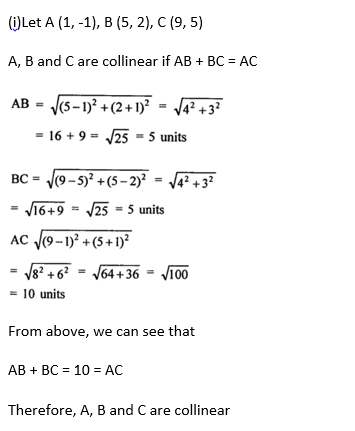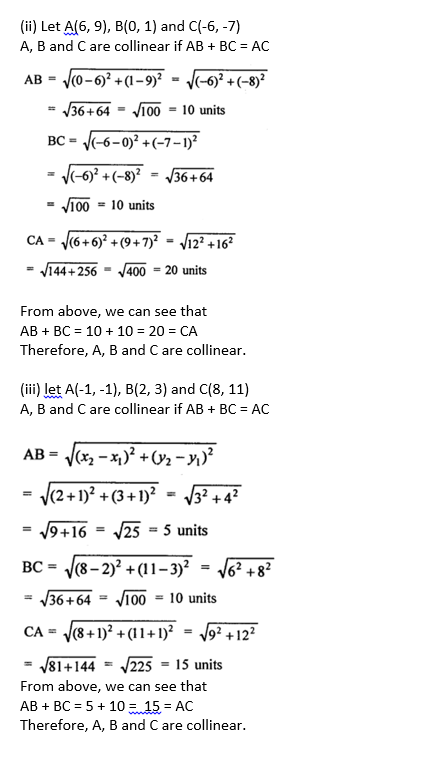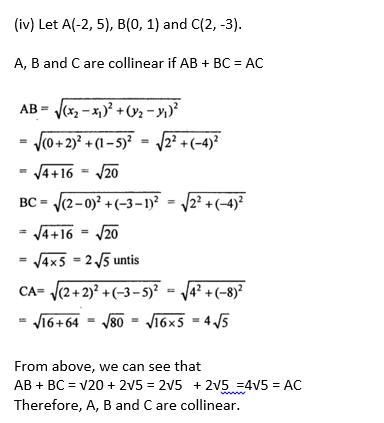Question 19: Show that the points A(7, 10), B(-2, 5) and C(3, -4) are the vertices of an isosceles right triangle.

Solution:

Given points are A(7, 10), B(-2, 5) and C(3, -4)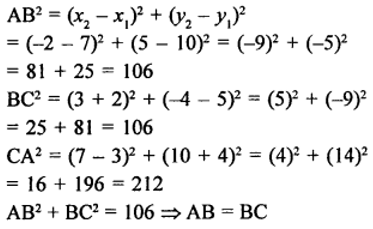From above, two of the sides are of equal length, so triangle ABC is an isosceles triangle.

Check for: Isosceles right triangle

Sum of square of two sides = Square of third side

AB^2 + BC^2 = 106 + 106 = 212 = CA^2

Hence given points are vertices of an isosceles right triangle.

Question 20: Show that the points A (3, 0), B (6, 4) and C (-1, 3) are the vertices of an isosceles right triangle.

Solution:

Given points are A (3, 0), B (6, 4) and C (-1, 3)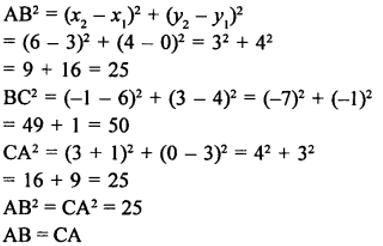From above, two of the sides are of equal length, so triangle ABC is an isosceles triangle.

Check for : Isosceles right triangle

Sum of square of two sides = Square of third side

AB^2 + AC^2 = 25 + 25 = 50 = CB^2

Hence given points are vertices of an isosceles right triangle.

## R S Aggarwal Solutions for Class 10 Maths Chapter 6 Coordinate Geometry Exercise 6A Topics

Class 10 Maths Chapter 6 Coordinate Geometry Exercise 6A is based on the following topics:

• Distance between two points:

The distance between two points A(x1, y1) and (x2, y2) is given by the formula: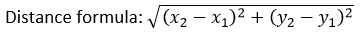• The distance of the point A(x, y) from the origin O(0,0) is given by: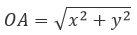• Points on Axes:

Any point on the x-axis is of the form (x, 0).

Any point on the y-axis is of the form (0, y).

• Properties of various types of quadrilaterals.
• Collinear Points: Three points are said to be collinear if they lie on the same straight line.